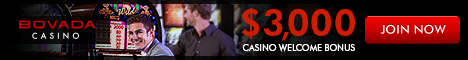HomeChoosing a CasinoBlackjack RulesBasic StrategyFree BlackjackBlackjack HistoryOnline Blackjack TipsBlackjack StrategyBlackjack TerminologyCard Counting  • Simple Counting • Standard Hi Lo • Shuffle TrackingOther BlackjackTournamentsLeer je Blackjack termen en speelDe beste Blackjack tips om te winnen What's Your Game? ------------------------ BlackJack Poker Roulette Video Poker# Blackjack Basic Strategy

When people refer to the 'basic strategy' for blackjack, they are speaking about a very specific thing. That is, a strategy card which tells you what the most profitable decision is for every possible hand you have showing, in relation to what the dealer is showing. These have been worked out with computer simulations that follow every descision through, and calculate what would be most profitable for the player. Blackjack basic strategy cards are specific to a couple of variables: the number of decks in the shoe, the rules the dealer must follow for standing and hitting, the players ability to double down (only on totals of 9/10, or any two cards?), players ability to double down after a split, and whether or not surrender is an option. Along the top of the chart are all of the possible dealer up-cards, then down the left hand side are all of the two-card combinations you could possible have. Follow the row that indicates your hand over to the column that is the dealer's up-card. In the cell you've just found there will be a letter indicating to either Hit, Stand, Split, or Double Down.
 2 3 4 5 6 7 8 9 T A Pair Splitting PAIRS Dealer's Upcard Your Cards (A,A) Y Y Y Y Y Y Y Y Y Y (T,T) N N N N N N N N N N (9,9) Y Y Y Y Y N Y Y N N (8,8) Y Y Y Y Y Y Y Y Y Y (7,7) Y Y Y Y Y Y Y N S N (6,6) Y Y Y Y Y Y N N N N (5,5) N N N N N N N N N N (4,4) N N Y Y Y N N N N N (3,3) Y Y Y Y Y Y Y N N N (2,2) Y Y Y Y Y Y N N N N Key: Y Yes, Split the pair. N No, Don't split the pair. S Stand

 Soft Totals SOFT TOTALS Dealer's Upcard Your Cards 2 3 4 5 6 7 8 9 T A (A,9) S S S S S S S S S S (A,8) S S S S Ds S S S S S (A,7) S Ds Ds Ds Ds S S H H S (A,6) D D D D D H H H H H (A,5) H H D D D H H H H H (A,4) H H D D D H H H H H (A,3) H H D D D H H H H H (A,2) H H D D D H H H H H Key: H Hit S Stand D Double if allowed; If not, hit. Ds Double if allowed; If not, stand.

 2 3 4 5 6 7 8 9 T A Hard Totals HARD TOTALS Dealer's Upcard Your Cards 17 S S S S S S S S S S 16 S S S S S H H H H H 15 S S S S S H H H H H 14 S S S S S H H H H H 13 S S S S S H H H H H 12 H H S S S H H H H H 11 D D D D D D D D D D 10 D D D D D D D D H H 9 D D D D D H H H H H 8 H H H D D H H H H H Key: H Hit S Stand D Double if allowed; If not, hit. Ds Double if allowed; If not, stand.